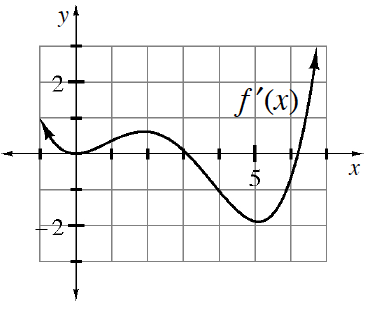### Home > APCALC > Chapter 9 > Lesson 9.4.2 > Problem9-120

9-120.

Use the graph of $y = f^\prime(x)$ at right to complete parts (a) through (e) below.

1. For what $x$-value(s) does $f$ have a relative minimum?

This occurs when $f^\prime(x)$ changes from negative to positive.

2. Where does $f$ have points of inflection?

This occurs when the slope of $f^\prime(x)$ is $0$.

3. Describe what happens on the graph of $y = f^{\prime\prime}(x)$ when $x = 5$.

What is the slope of the given graph at $x = 4.8$?
At $x = 5$?
At $x = 5.2$?

4. Describe the graph of $y = f(x)$ when $x = 0$.

5. If $f(0) = 2$, estimate $f(3)$.
$f(3)=f(0)+\int_0^3f^\prime(x)dx$